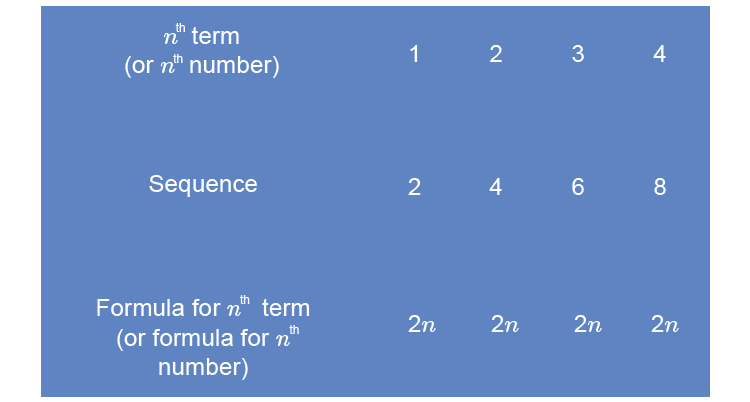# The N^(th) term of a sequence

The word term is difficult to understand because term can mean:

• a number
• a variable
• a number times one or more variables

So term could be 6 or y or 3xy, but it is usually in a formula between a +ve and a -ve sign as:

5x+3+8y

(See our Mammoth memory definition of term)

However, in sequences term means number.

To remember what the n^(th) term is, remember one simple example and then the others will become obvious.NOTE:

This sequence 2, 4, 6, 8 has to be one of the most famous sequences ever.